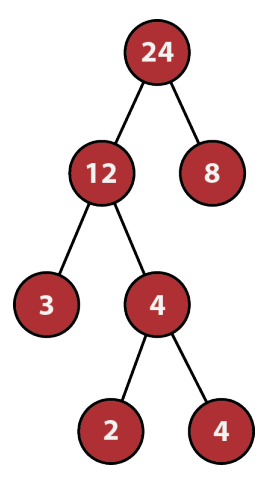시간 제한 메모리 제한 제출 정답 맞은 사람 정답 비율
2 초 512 MB 0 0 0 0.000%

문제An LCM tree is a binary tree in which each node has a positive integer value, and either zero or two children. If two nodes x and y are the children of node z, then the Least Common Multiple (LCM) of the values of node x and node y must equal the value of node z.

You are given n nodes with positive integer values to be arranged into an LCM tree. In how many ways (modulo 109 + 7) can you do that? Two ways are considered different if there are two nodes x and y so that x is a child of y in one way but not in the other way.

The illustration shows one of the two ways for the first sample case. The other way can be obtained by swapping the two nodes with value 4. Note that swapping the two leaves with values 2 and 4 does not give a different way.

입력

The first line has an odd integer n (1 ≤ n ≤ 25). The second line has n positive integers no larger than 109, giving the values of the nodes.

출력

Output the number of ways to arrange the given nodes into an LCM tree, modulo 109 + 7.

예제 입력 1

7
2 3 4 4 8 12 24


예제 출력 1

2


예제 입력 2

3
7 7 7


예제 출력 2

3


예제 입력 3

5
1 2 3 2 1


예제 출력 3

0


예제 입력 4

13
1 1 1 1 1 1 1 1 1 1 1 1 1


예제 출력 4

843230316